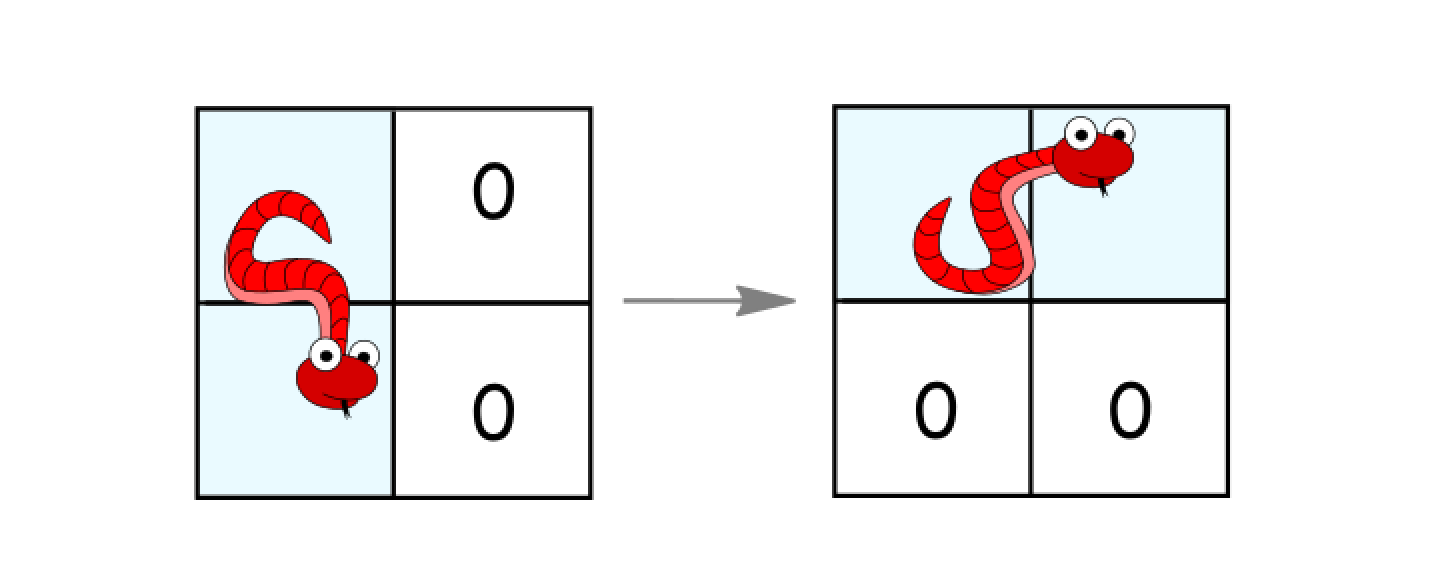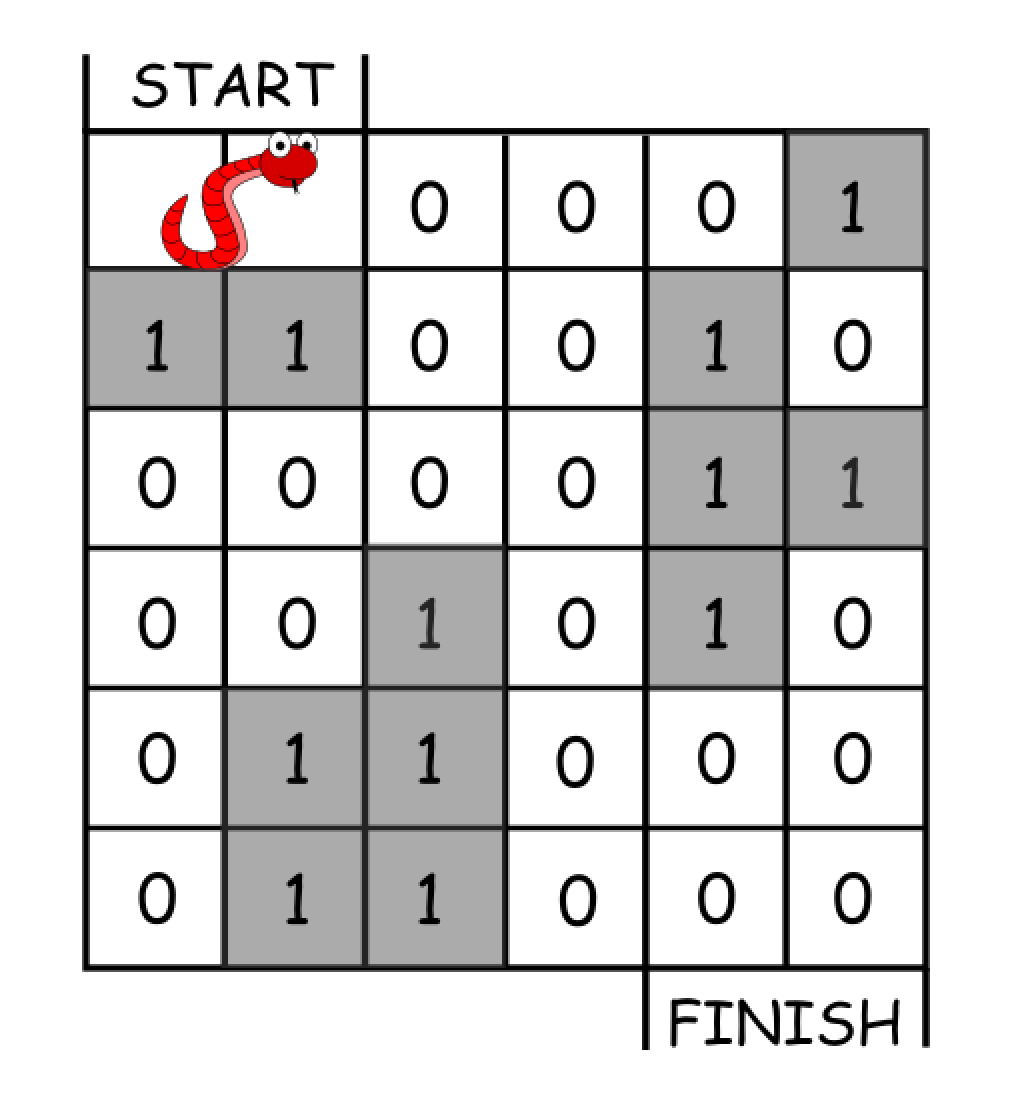Formatted question description: https://leetcode.ca/all/1210.html

# 1210. Minimum Moves to Reach Target with Rotations (Hard)

In an n*n grid, there is a snake that spans 2 cells and starts moving from the top left corner at (0, 0) and (0, 1). The grid has empty cells represented by zeros and blocked cells represented by ones. The snake wants to reach the lower right corner at (n-1, n-2) and (n-1, n-1).

In one move the snake can:

• Move one cell to the right if there are no blocked cells there. This move keeps the horizontal/vertical position of the snake as it is.
• Move down one cell if there are no blocked cells there. This move keeps the horizontal/vertical position of the snake as it is.
• Rotate clockwise if it's in a horizontal position and the two cells under it are both empty. In that case the snake moves from (r, c) and (r, c+1) to (r, c) and (r+1, c).• Rotate counterclockwise if it's in a vertical position and the two cells to its right are both empty. In that case the snake moves from (r, c) and (r+1, c) to (r, c) and (r, c+1).Return the minimum number of moves to reach the target.

If there is no way to reach the target, return -1.

Example 1:Input: grid = [[0,0,0,0,0,1],
[1,1,0,0,1,0],
[0,0,0,0,1,1],
[0,0,1,0,1,0],
[0,1,1,0,0,0],
[0,1,1,0,0,0]]
Output: 11
Explanation:
One possible solution is [right, right, rotate clockwise, right, down, down, down, down, rotate counterclockwise, right, down].


Example 2:

Input: grid = [[0,0,1,1,1,1],
[0,0,0,0,1,1],
[1,1,0,0,0,1],
[1,1,1,0,0,1],
[1,1,1,0,0,1],
[1,1,1,0,0,0]]
Output: 9


Constraints:

• 2 <= n <= 100
• 0 <= grid[i][j] <= 1
• It is guaranteed that the snake starts at empty cells.

Related Topics:

## Solution 1. BFS

Since we are looking for the shortest path, BFS is our first option.

// OJ: https://leetcode.com/problems/minimum-moves-to-reach-target-with-rotations/

// Time: O(N^2)
// Space: O(N^2)
class Solution {
public:
int minimumMoves(vector<vector<int>>& G) {
queue<int> q;
q.push(0);
unordered_set<int> seen;
seen.insert(0);
int step = 0, N = G.size();
auto safe = [&](int p) {
int y = p % 1000, x = p / 1000 % 1000, dir = p / 1000000;
if (x < 0 || y < 0 || x >= N || y >= N || G[x][y] == 1) return false;
return (dir == 0 && y + 1 < N && G[x][y + 1] == 0)
|| (dir == 1 && x + 1 < N && G[x + 1][y] == 0);
};
while (q.size()) {
int cnt = q.size();
while (cnt--) {
int p = q.front(), y = p % 1000, x = p / 1000 % 1000, dir = p / 1000000;
if (x == N - 1 && y == N - 2) return step;
q.pop();
// move right
int r = p + 1;
if (seen.count(r) == 0 && safe(r)) {
seen.insert(r);
q.push(r);
}
// move down
int d = p + 1000;
if (seen.count(d) == 0 && safe(d)) {
seen.insert(d);
q.push(d);
}
// rotate closewise
if (dir == 0) {
int p2 = 1000000 + x * 1000 + y, cx = x + 1, cy = y + 1;
if (seen.count(p2) || !safe(p2) || cx < 0 || cy < 0 || cx >= N || cy >= N || G[cx][cy] == 1) continue;
seen.insert(p2);
q.push(p2);
}
// rotate counterclosewise
if (dir == 1) {
int p2 = x * 1000 + y, cx = x + 1, cy = y + 1;
if (seen.count(p2) || !safe(p2) || cx < 0 || cy < 0 || cx >= N || cy >= N || G[cx][cy] == 1) continue;
seen.insert(p2);
q.push(p2);
}
}
++step;
}
return -1;
}
};


Java

class Solution {
public int minimumMoves(int[][] grid) {
final int BLOCK = -1;
final int WHITE = 0;
final int GRAY = 1;
final int BLACK = 2;
int rows = grid.length, columns = grid.length;
if (grid[rows - 1][columns - 1] == 1 || grid[rows - 1][columns - 2] == -1)
return -1;
int[][][] colors = new int[rows][columns];
int[][][] distances = new int[rows][columns];
for (int i = 0; i < rows; i++)
colors[i][columns - 1] = BLOCK;
for (int i = 0; i < columns; i++)
colors[rows - 1][i] = BLOCK;
for (int i = 0; i < rows; i++) {
for (int j = 0; j < columns; j++) {
if (grid[i][j] == 1) {
colors[i][j] = BLOCK;
colors[i][j] = BLOCK;
if (i > 0)
colors[i - 1][j] = BLOCK;
if (j > 0)
colors[i][j - 1] = BLOCK;
}
distances[i][j] = Integer.MAX_VALUE;
distances[i][j] = Integer.MAX_VALUE;
}
}
colors = GRAY;
distances = 0;
queue.offer(new int[]{0, 0, 0});
while (!queue.isEmpty()) {
int[] status = queue.poll();
int row = status, column = status, direction = status;
int distance = distances[row][column][direction];
int newRow = row + 1, newColumn = column + 1, newDirection = 1 - direction;
int newDistance = distance + 1;
if (newRow < rows && colors[newRow][column][direction] == WHITE) {
colors[newRow][column][direction] = GRAY;
distances[newRow][column][direction] = newDistance;
queue.offer(new int[]{newRow, column, direction});
}
if (newColumn < columns && colors[row][newColumn][direction] == WHITE) {
colors[row][newColumn][direction] = GRAY;
distances[row][newColumn][direction] = newDistance;
queue.offer(new int[]{row, newColumn, direction});
}
if (colors[row][column][newDirection] == WHITE) {
if (row < rows - 1 && column < columns - 1 && grid[row + 1][column + 1] == 0) {
colors[row][column][newDirection] = GRAY;
distances[row][column][newDirection] = newDistance;
queue.offer(new int[]{row, column, newDirection});
}
}
colors[row][column][direction] = BLACK;
}
int totalMoves = distances[rows - 1][columns - 2];